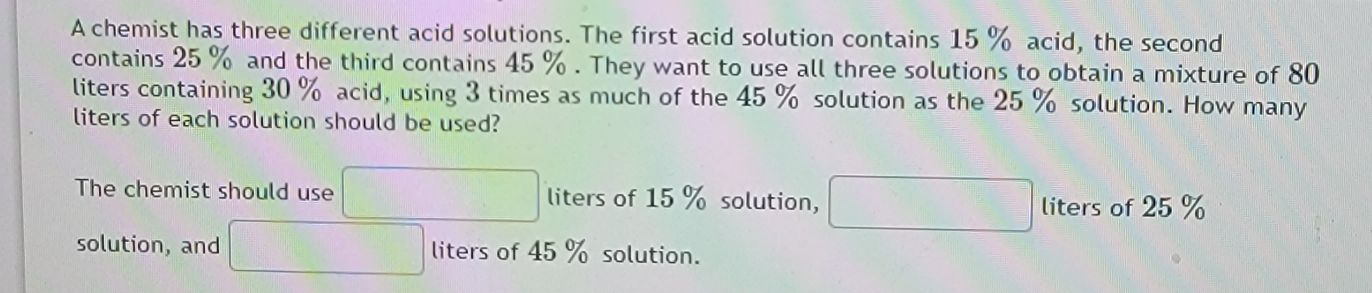### ¿Todavía tienes preguntas de matemáticas?

Pregunte a nuestros tutores expertos
Algebra
PreguntaA chemist has three different acid solutions. The first acid solution contains $$15 \%$$ acid, the second contains $$25 \%$$ and the third contains $$45 \%$$ . They want to use all three solutions to obtain a mixture of $$80$$ liters containing $$30 \%$$ acid, using $$3$$ times as much of the $$45 \%$$ solution as the $$25 \%$$ solution. How many liters of each solution should be used?